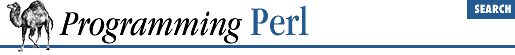home | O'Reilly's CD bookshelfs | FreeBSD | Linux | Cisco | Cisco Exam

#### 3.2.21 cos

```cos ```

EXPR
```
```

This function returns the cosine of ``` EXPR ``` (expressed in radians). For example, the following script will print a cosine table of angles measured in degrees:

```# Here's the lazy way of getting degrees-to-radians.

\$pi = atan2(1,1) * 4;
\$piover180 = \$pi/180;

# Print table.

for (\$_ = 0; \$_ <= 90; \$_++) {
printf "%3d %7.5f\n", \$_, cos(\$_ * \$piover180);
}```

For the inverse cosine operation, you may use the ``` POSIX::acos()``` function, or use this relation:

`sub acos { atan2( sqrt(1 - \$_ * \$_), \$_ ) }`